# Sierpinski Fractals

## Introduction

There are quite a lot of fractals named after Waclaw Sierpinski, a Polish mathematician who lived from 1882 to 1969.

These include the Sierpinski Triangle, the Sierpinski Carpet, the Sierpinski Pyramid (the 3D version of the Sierpinski Triangle) and the Sierpinski Cube (the 3D version of the Sierpinski Carpet). The 2D figures will be described here.

## Sierpinski Triangle

The Sierpinski Triangle, also called Sierpinski Gasket and Sierpinski Sieve, can be drawn by hand as follows:

Start with a single triangle. This is the only triangle in this direction, all the others will be upside down: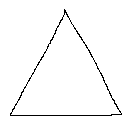Inside this triangle, draw a smaller upside down triangle. Its corners should be exactly in the centers of the sides of the large triangle: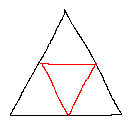Now, draw 3 smaller triangles in each of the 3 triangles that are pointing upwards, again with the corners in the centers of the sides of the triangles that point upwards: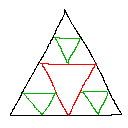Now there are 9 triangles pointing upwards. In each of these 9, draw again smaller upside down triangles: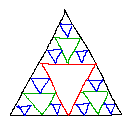In the 27 triangles pointing upwards, again draw 27 triangles pointing downwards: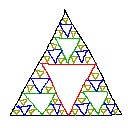Rinse, repeat.

After infinite steps, and if all triangles pointing upwards would be filled, you have the Sierpinski Sieve.

Every step, more triangles have to be drawn. This is a recursive process, and it can be drawn the same way with a computer.

### With Recursion

We'll now program that what was drawn by hand on the computer, by making a triangle drawing function that'll call itself again 3 times, until n steps of recursions are reached. This program is made so that it works for any initial triangle, it doesn't have to be symmetrical, the only condition is that the corners lie inside the screen.

The main function sets up the screen and calls the drawSierpinski function. The drawSierpinski function itself will draw only one triangle: the initial one that points upwards. Then it'll call the function subTriangle, and that is the actual recursive function, that'll draw all the upside down triangles.

The function subTriangle draws a single upside down triangle, with the 3 corners you give it with its parameters. Then it'll call itself 3 times again, to draw 3 smaller triangles. For these 3 triangles, new corners are used of course, and these have to be calculated. In the following image, if the black triangle is the big triangle the subTriangle function has drawn, then the 3 red triangles are the new ones that have to be calculated: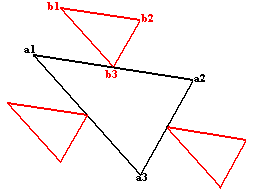The corners of the big triangle are a1, a2 and a3. The corners of one of the smaller triangles are b1, b2 and b3 as you can see on the image. If we see all these points as vectors, the the formulas for the points b (with the points a being known) are:

b3 = (a1 + a2) / 2, because b3 lies in the center between a1 and a2, this point is the average of a1 and a2!

b1 = b3 + (a1 - a3) / 2: if you examine well, you'll see that the point b1 is the sum of the point b3 and the vector (a1 - a3) / 2, it's divided through 2 because the corresponding side of the smaller triangle is half as big.

And finally,

b2 = b3 + (a2 - a3) / 2: this is very similar to the other formula, but with the other side.

For the other 2 small triangles, something similar is done.

In the code, we aren't using a vector class, so x and y are separate variables, and because of the way vector additions work, we simply have to do the same thing twice, once for x, and once for y, with the same formulas.

For the coordinates of triangle corners, floating point numbers are used for more precision.

With this knowledge the program can be made, the comments in the code below will explain how it works:

 ```//Declaration of the drawSierpinski function. The coordinates are the 3 outer corners of the Sierpinski Triangle. void drawSierpinski(float x1, float y1, float x2, float y2, float x3, float y3); //Declaration of the subTriangle function, the coordinates are the 3 corners, and n is the number of recursions. void subTriangle(int n, float x1, float y1, float x2, float y2, float x3, float y3); //depth is the number of recursive steps int depth = 7; //The main function sets up the screen and then calls the drawSierpinski function int main(int argc, char *argv[]) { screen(640, 480, 0, "Sierpinski Triangle"); cls(RGB_White); //Make the background white drawSierpinski(10, h - 10, w - 10, h - 10, w / 2, 10); //Call the sierpinski function (works with any corners inside the screen) //After drawing the whole thing, redraw the screen and wait until the any key is pressed redraw(); sleep(); return(0); } //This function will draw only one triangle, the outer triangle (the only not upside down one), and then start the recursive function void drawSierpinski(float x1, float y1, float x2, float y2, float x3, float y3) { //Draw the 3 sides of the triangle as black lines drawLine(int(x1), int(y1), int(x2), int(y2), RGB_Black); drawLine(int(x1), int(y1), int(x3), int(y3), RGB_Black); drawLine(int(x2), int(y2), int(x3), int(y3), RGB_Black); //Call the recursive function that'll draw all the rest. The 3 corners of it are always the centers of sides, so they're averages subTriangle ( 1, //This represents the first recursion (x1 + x2) / 2, //x coordinate of first corner (y1 + y2) / 2, //y coordinate of first corner (x1 + x3) / 2, //x coordinate of second corner (y1 + y3) / 2, //y coordinate of second corner (x2 + x3) / 2, //x coordinate of third corner (y2 + y3) / 2 //y coordinate of third corner ); } //The recursive function that'll draw all the upside down triangles void subTriangle(int n, float x1, float y1, float x2, float y2, float x3, float y3) { //Draw the 3 sides as black lines drawLine(int(x1), int(y1), int(x2), int(y2), RGB_Black); drawLine(int(x1), int(y1), int(x3), int(y3), RGB_Black); drawLine(int(x2), int(y2), int(x3), int(y3), RGB_Black); //Calls itself 3 times with new corners, but only if the current number of recursions is smaller than the maximum depth if(n < depth) { //Smaller triangle 1 subTriangle ( n+1, //Number of recursions for the next call increased with 1 (x1 + x2) / 2 + (x2 - x3) / 2, //x coordinate of first corner (y1 + y2) / 2 + (y2 - y3) / 2, //y coordinate of first corner (x1 + x2) / 2 + (x1 - x3) / 2, //x coordinate of second corner (y1 + y2) / 2 + (y1 - y3) / 2, //y coordinate of second corner (x1 + x2) / 2, //x coordinate of third corner (y1 + y2) / 2 //y coordinate of third corner ); //Smaller triangle 2 subTriangle ( n+1, //Number of recursions for the next call increased with 1 (x3 + x2) / 2 + (x2 - x1) / 2, //x coordinate of first corner (y3 + y2) / 2 + (y2 - y1) / 2, //y coordinate of first corner (x3 + x2) / 2 + (x3 - x1) / 2, //x coordinate of second corner (y3 + y2) / 2 + (y3 - y1) / 2, //y coordinate of second corner (x3 + x2) / 2, //x coordinate of third corner (y3 + y2) / 2 //y coordinate of third corner ); //Smaller triangle 3 subTriangle ( n+1, //Number of recursions for the next call increased with 1 (x1 + x3) / 2 + (x3 - x2) / 2, //x coordinate of first corner (y1 + y3) / 2 + (y3 - y2) / 2, //y coordinate of first corner (x1 + x3) / 2 + (x1 - x2) / 2, //x coordinate of second corner (y1 + y3) / 2 + (y1 - y2) / 2, //y coordinate of second corner (x1 + x3) / 2, //x coordinate of third corner (y1 + y3) / 2 //y coordinate of third corner ); } }```

Here's what you see after running the program: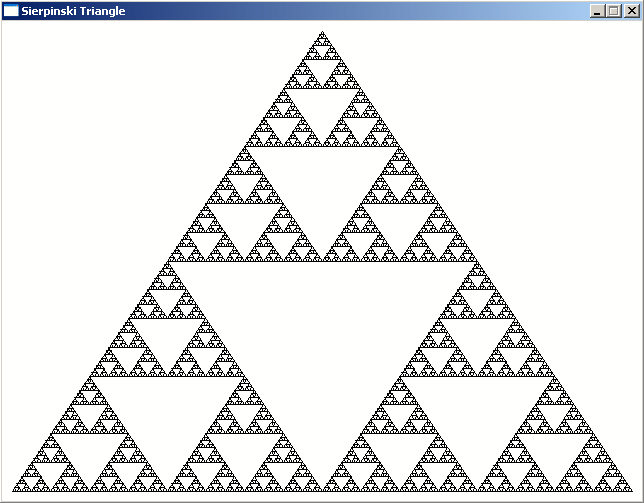### With "AND"

The method given above is only one of the very many ways to draw Sierpinski Triangles. One of these ways is with the AND operator. If you take of a pixel the x-coordinate as an integer, and the y-coordinate as an integer, and use the AND operator on them, if the result is 0, draw a pixel of one color, otherwise another. You'll then see a Sierpinski Triangle pop up!

The AND operator on two integers, takes both integers as a binary number, and uses AND on each of the corresponding bits. The AND operator on bits works as follows::

 Operation Result 0 AND 0 0 0 AND 1 0 1 AND 0 0 1 AND 1 1

This is done for every bit of the integer, and only if the resulting integer is in binary 0000000000000000, the pixel is given another color.

The code is a very simple double loop that goes through every pixel and checks if x & y is 0 or not, where "&" is the binary AND operator in C++. By using "x & y" inside an if condition, it'll only be false in the case x & y is 0, and in all other cases a white pixel is drawn, so the result will be a black sierpinski triangle on a white background.

 ```int main(int argc, char *argv[]) { screen(256, 256, 0, "AND"); for(int y = 0; y < h; y++) for(int x = 0; x < w; x++) { if(x & y) pset(x, y, RGB_White); } redraw(); sleep(); return 0; }```

The result looks best if the screen sizes are powers of 2, otherwise you see only a part of the triangle.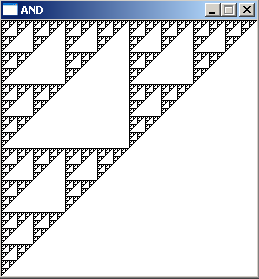### With a Random Function

Another totally different way to draw an approximation of the Sierpinski Triangle works as follows:

1) define 3 points with coordinates: a (ax, ay), b (bx, by) and c (cx, cy). These will become the corners of the large outer triangle.

2) define another point, p (px, py) and place it in a corner of the triangle (for example px = ax and py = ay).

3) draw a point at location (px, py) with a pencil

4) roll a theoretical die with 3 sides (side 0, side 1 and side 2), or just make a random choice between "0", "1" and "2".

5) now change the coordinates of point p, depending on what number you rolled:
• if you rolled 0, p = (p+a) / 2
• if you rolled 1, p = (p+b) / 2
• if you rolled 2, p = (p+c) / 2
6) go back to step 2, there place the point at the new position of p, and keep looping through this until you get tired

Even though the whole process is randomized, after enough steps, the result will look more and more like a Sierpinski Triangle!

This isn't too hard to code, the comments explain how everything works:

 ```//How much pixels should be randomly chosen and drawn #define numSteps 10000 int main(int argc, char *argv[]) { //create the screen and make it white screen(256, 256, 0, "Sierpinski Triangle"); cls(RGB_White); //the 3 corners of the outer triangle float ax = 10; float ay = h - 10; float bx = w - 10; float by = h - 10; float cx = w / 2; float cy = 10; //initial coordinates for the point px float px = ax; float py = ay; //do the process numSteps times for(int n = 0; n < numSteps; n++) { //draw the pixel pset(int(px), int(py), RGB_Black); //pick a random number 0, 1 or 2 switch(abs(rand() % 3)) { //depending on the number, choose another new coordinate for point p case 0: px = (px + ax) / 2.0; py = (py + ay) / 2.0; break; case 1: px = (px + bx) / 2.0; py = (py + by) / 2.0; break; case 2: px = (px + cx) / 2.0; py = (py + cy) / 2.0; break; } } //redraw, sleep and end the program redraw(); sleep(); return(0); }```

The bigger you make the numSteps value, the more pixels will be drawn. These images show the result for 1000, 10000 and 100000 steps: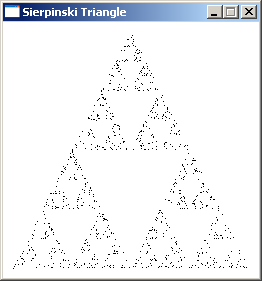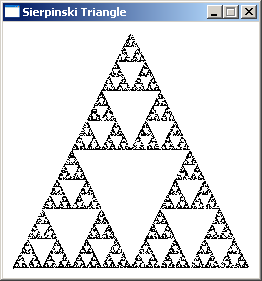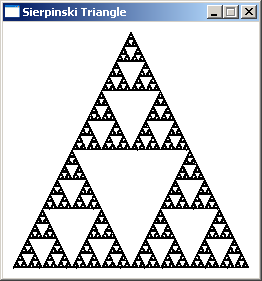By playing a bit with the formulas you can get other shapes, like for example this one: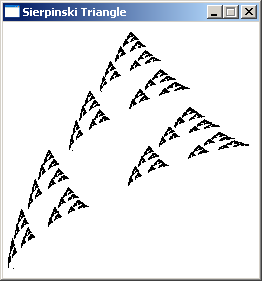Gotten by changing some of the divisions through 2.0 into divisions through 3.0.

### With Rectangle Recursion

Yet another way to draw a Sierpinski Triangle is with a recursive function that uses rectangles. The recursion process works as follows: Change every rectangle into an L-shape:The L-shape itself consists out of 3 rectangles, which are again converted into an L-shape, etc...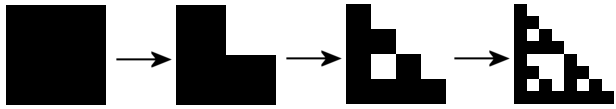If you keep doing this long enough, it'll look more and more like a Sierpinski Triangle. This is again something that can be programmed with a recursive function, pretty similar to the recursive code given higher, but this times rectangles are drawn, and new coordinates for 3 new rectangles have to be calculated every time.

 ```//the coordinates are 2 corners of the rectangle, n is the current recursion step void drawSierpinski(int n, int x1, int y1, int x2, int y2); //how much recursions maximum (after log_2(screenWidth) recursions the rectangles are smaller than a pixel) #define maxRecursions 8 int main(int argc, char *argv[]) { //create the screen and make it white screen(256, 256, 0, "Sierpinski Triangle"); //start the recursive function drawSierpinski(1, 0, 0, w - 1, h - 1); //redraw, sleep, etc... redraw(); sleep(); return(0); } void drawSierpinski(int n, int x1, int y1, int x2, int y2) { //draw white rectangle in the upper right part, thereby making a black L drawRect((x1 + x2) / 2, y1, x2 - 1, (y1 + y2) / 2 - 1, RGB_White); //call itself 3 times again, now for the 3 new rectangles in the L shape if(n < maxRecursions) { drawSierpinski(n + 1, x1, y1, (x1 + x2) / 2, (y1 + y2) / 2); drawSierpinski(n + 1, x1, (y1 + y2) / 2, (x1 + x2) / 2, y2); drawSierpinski(n + 1, (x1 + x2) / 2, (y1 + y2) / 2, x2, y2); } }```

Here's the result for 3, 5 and 8 recursions: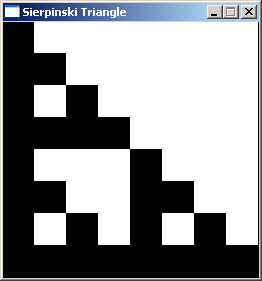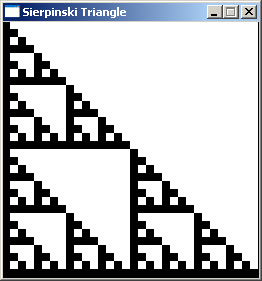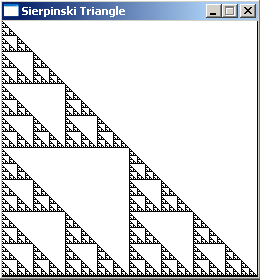To study the recursion a bit better, try to see what happens when the drawSierpinski function calls itself only 1 time instead of 3 (the other 2 calls are commented out): now, if maxRecursions is 8, only 8 rectangles are drawn, each one smaller than the previous one: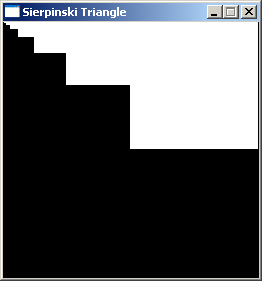Now, we enable two of the calls to itself, and already more squares are being drawn, but the shape isn't so complex yet. Now on the right is 1 big square, left of it 2 half squares, left of those 4 1/4th squares, left of that 8 1/8th squares, and so on, with to the leftmost row 128 squares that are 128 times smaller than the big square on the right.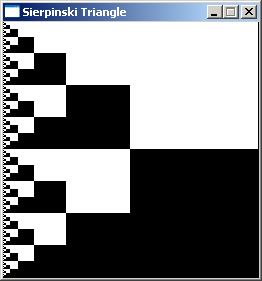And finally, if we enable all 3 calls to itself, we get the Sierpinski Triangle, and so many squares are drawn that almost everything is white:There's even another way to get a Sierpinski Triangle: with a cellular automaton, but this might be covered in a later article.

## Sierpinski Carpet

### With Rectangle Recursion

A different fractal is the Sierpinski Carpet. To draw one by hand, start with a white square, and then draw a black square in the center with each side 1/3th of the size of the original square: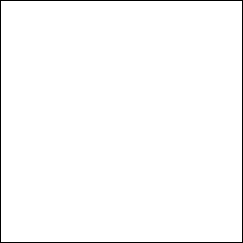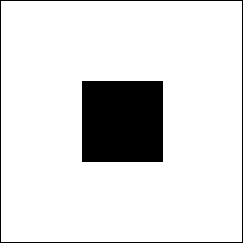Now, around the black square, are 8 white squares. In each of these 8, draw again a black square that is 1/8th smaller, and in the 8*8=64 new white squares, do it yet again: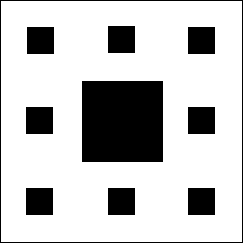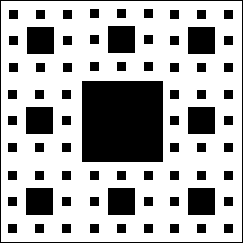Keep doing this until infinity, and you get a sierpinski carpet!

To draw it, we can use a recursive function that's pretty similar to the one used for the sierpinski triangle with rectangles, but now the function has to call itself 8 times instead of only 3, and use different coordinates. The coordinates x1,y1-x2,y2 get divided in 9 sections, in the center one the rectangle is drawn with rect, and the other 8 ones are used as parameters for the drawCarpet calls of the next recursion step.

 ```//the coordinates are 2 corners of the rectangle, n is the current recursion step void drawCarpet(int n, float x1, float y1, float x2, float y2); //how much recursions maximum #define maxRecursions 6 int main(int argc, char *argv[]) { //create the screen and make it white, use powers of 3 for the screen size for best result screen(243, 243, 0, "Sierpinski Carpet"); cls(RGB_White); //start the recursive function drawCarpet(1, 0, 0, w - 1, h - 1); //redraw, sleep, etc... redraw(); sleep(); return(0); } void drawCarpet(int n, float x1, float y1, float x2, float y2) { //draw black rectangle with 1/3th the size in the center of the given coordinates drawRect(int((2 * x1 + x2) / 3.0), int((2 * y1 + y2) / 3.0), int((x1 + 2 * x2) / 3.0) - 1, int((y1 + 2 * y2) / 3.0) - 1, RGB_Black); //call itself 8 times again, now for the 8 new rectangles around the one that was just drawn if(n < maxRecursions) { drawCarpet(n + 1, x1 , y1 , (2 * x1 + x2) / 3.0, (2 * y1 + y2) / 3.0); drawCarpet(n + 1, (2 * x1 + x2) / 3.0, y1 , (x1 + 2 * x2) / 3.0, (2 * y1 + y2) / 3.0); drawCarpet(n + 1, (x1 + 2 * x2) / 3.0, y1 , x2 , (2 * y1 + y2) / 3.0); drawCarpet(n + 1, x1 , (2 * y1 + y2) / 3.0, (2 * x1 + x2) / 3.0, (y1 + 2 * y2) / 3.0); drawCarpet(n + 1, (x1 + 2 * x2) / 3.0, (2 * y1 + y2) / 3.0, x2 , (y1 + 2 * y2) / 3.0); drawCarpet(n + 1, x1 , (y1 + 2 * y2) / 3.0, (2 * x1 + x2) / 3.0, y2 ); drawCarpet(n + 1, (2 * x1 + x2) / 3.0, (y1 + 2 * y2) / 3.0, (x1 + 2 * x2) / 3.0, y2 ); drawCarpet(n + 1, (x1 + 2 * x2) / 3.0, (y1 + 2 * y2) / 3.0, x2 , y2 ); } }```

And here's the result for 6 recursion steps: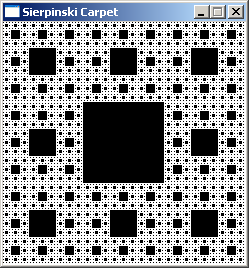If you want a bigger one, use a resolution of 729*729 pixels and 7 recursions.

### With Ternary Numbers

There's also a method to draw the Sierpinski Carpet that's similar to the "AND" method for drawing the sierpinski triangle. Namely, you do a calculation for each pixel to check whether or not it should be colored.

This method is a bit more complex though, because you have to work in base 3, i.e. with ternary numbers. Ternary numbers have digits that can be '0', '1' or '2'.

The method works as follows:

Take both the coordinates of the pixel as integers written in ternary notation. For every digit, check if NOT both corresponding digits are '1'. If this never happens, then the point belongs to the carpet.

To find the first (rightmost) ternary digit of a number, modulo divide it though 3 (modulo division through 3 works as follows: 0%3=0, 1%3=1, 2%3=2, 3%3=0, 4%3=1, 5%3=2, 6%3=0, 7%3=1, etc.....).
To find the second ternary digit, first divide the number through 3 (integer division, i.e. remove the numbers behind the point), and then modulo divide it though 3.
To find the third ternary digit, divide it through 9, then modulo divide through 3.
To find the fourth, divide through 27, then modulo divide through 3,
etc...

The example below uses a resolution of 243 by 243 pixels, so we have to check only the first 5 ternary digits of the pixel coordinates, since with 5 ternary digits you can represent all numbers from 0 to 242.

The condition in the "if", is written out on many lines, and the condition checks for each of the 5 digits if NOT both the one from the x coordinate and the one from the y coordinate are together 1. If the condition is true, a white pixel is drawn at x,y.

 ```int main(int argc, char *argv[]) { screen(243, 243, 0, "Sierpinski Carpet"); for(int y = 0; y < h; y++) for(int x = 0; x < w; x++) { if ( //Not both the first (rightmost) digits are '1' in base 3 !( (x / 1) % 3 == 1 && (y / 1) % 3 == 1 ) && //Not both the second digits are '1' in base 3 !( (x / 3) % 3 == 1 && (y / 3) % 3 == 1 ) && //Not both the third digits are '1' in base 3 !( (x / 9) % 3 == 1 && (y / 9) % 3 == 1 ) && //Not both the fourth digits are '1' in base 3 !( (x / 27) % 3 == 1 && (y / 27) % 3 == 1 ) && //Not both the fifth digits are '1' in base 3 !( (x / 81) % 3 == 1 && (y / 81) % 3 == 1 ) ) pset(x, y, RGB_White); } redraw(); sleep(); return(0); }```

The result looks exactly the same as from the previous program, even though this one uses a totally different method: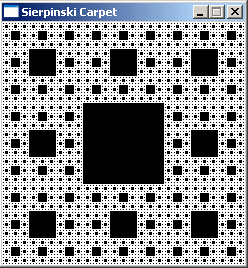If you want to increase the resolution, you have to add an extra condition for the 6th digit (where you divide through 243), etc... for every time you triple the resolution.

The condition that checks for the 5th digit (the 5th ternary digit from the right) is the one that's responsible for the big black square in the center.
The condition that checks for the 4th digit, is the one responsible for the 8 smaller squares around the center square,
etc...

If you remove the condition for the 5th digit, the center black square is gone, and instead you get this: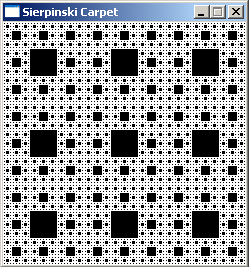If you remove instead for example the condition that checks for the 3th digit, all the black squares of the third order are gone while all others are left: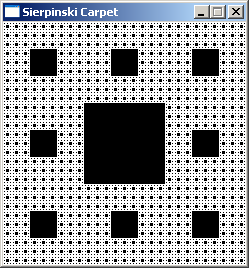So if you triple the resolution, the square that is now the center square will become a square in the top left, and you need an even bigger black square in the new center, which is why you need a new condition that checks for the 6th digit then.

Last edited: 2004